# 14-1100/Homework Assignment 1

This assignment is due at class time on Monday, October 6, 2011.

### Part I

Web search "Rubik's Cube Variants" (look at images), or look at Wikipedia: Combination Puzzle or TwistyPuzzles.com, or search elsewhere or go to a toy shop, pick your favourite "permutation group puzzle" (other than the Rubik Cube, of course), and figure out how many configurations it has. For your solution to count the number of configurations must be more than you can count, and your solution must include a clear picture or diagram of the object being studied, its labeling by integers, the list of generating permutations for it, and a printout of the program you used along with screen shot of its output (or an input/output log). It is ok to use the program presented in class (Mathematica is available on a departmental server; look for it!) but better to write your own. You can submit your solution either as a wiki page on this server (best option), or as a URL elsewhere (second best), or as a single file in any reasonable format, or on paper.

### Part II

Solve the following questions.

1. (Selick) If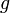$g$ is an element of a group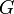$G$, the order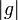$|g|$ of$g$ is the least positive number n for which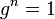$g^n=1$ (may be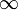$\infty$). If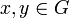$x,y\in G$, prove that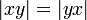$|xy|=|yx|$.
2. (Selick) Let$G$ be a group. Show that the function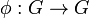$\phi:G\to G$ given by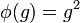$\phi(g)=g^2$ is a morphism of groups if and only if$G$ is Abelian.
3. (Lang, pp 75) Let$G$ be a group. For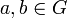$a,b\in G$, the commutator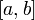$[a,b]$ of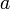$a$ and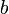$b$ is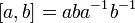$[a,b]=aba^{-1}b^{-1}$. Let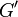$G'$ be the subgroup of$G$ generated by all commutators of elements of$G$. Show that$G'$ is normal in$G$, that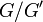$G/G'$ is Abelian, and that any morphism from$G$ into an Abelian group factors through$G/G'$.
4. (Lang, pp 75) Let$G$ be a group. An automorphism of$G$ is an invertible group morphism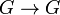$G\to G$. An inner automorphism is an automorphism of$G$ given by conjugation by some specific element$g$ of$G$, so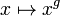$x\mapsto x^g$. Prove that the inner automorphisms of$G$ form a normal subgroup of the group of all automorphisms of$G$.

### Part III

After September 25, identify yourself in the 14-1100/Class Photo page! It is best (though not mandatory) if you do that on the 14-1100/Class Photo page itself.